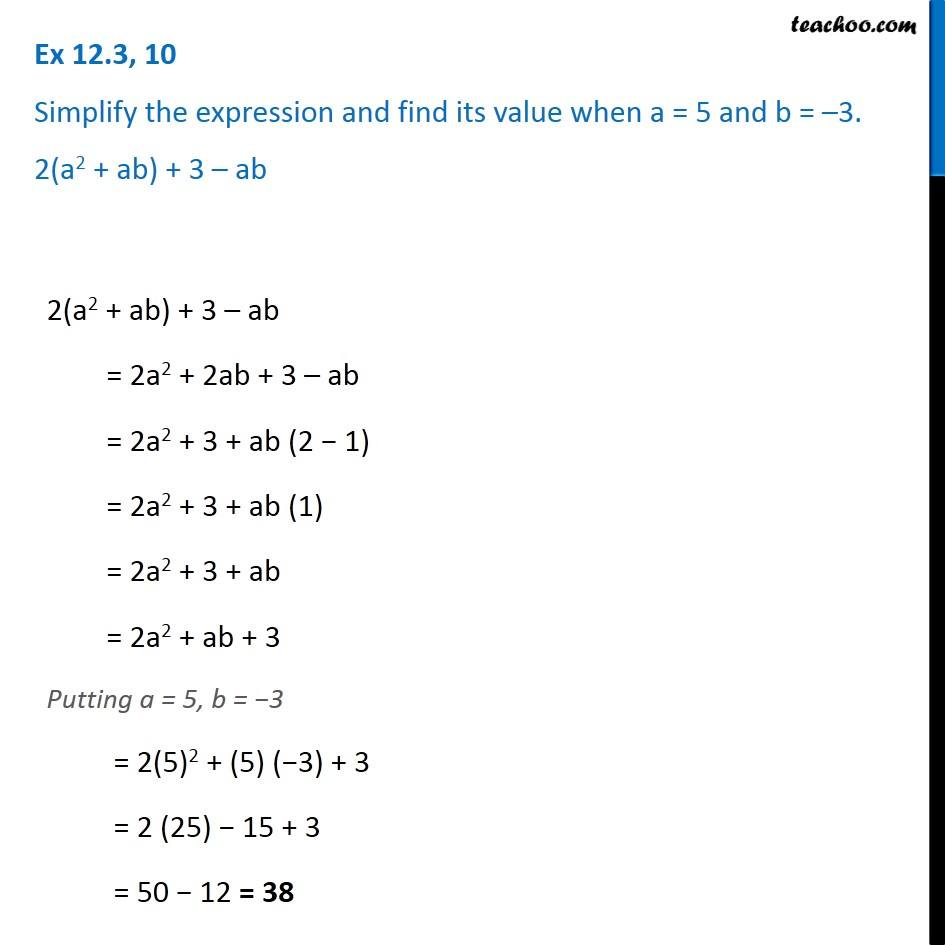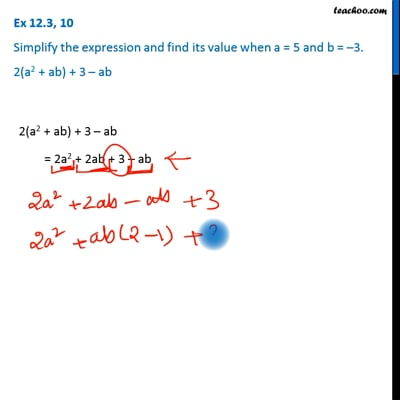Ex 12.3

Chapter 12 Class 7 Algebraic Expressions
Serial order wiseThis video is only available for Teachoo black users

Get live Maths 1-on-1 Classs - Class 6 to 12

### Transcript

Ex 12.3, 10 Simplify the expression and find its value when a = 5 and b = –3. 2(a2 + ab) + 3 – ab 2(a2 + ab) + 3 – ab = 2a2 + 2ab + 3 – ab = 2a2 + 3 + ab (2 − 1) = 2a2 + 3 + ab (1) = 2a2 + 3 + ab = 2a2 + ab + 3 Putting a = 5, b = −3 = 2(5)2 + (5) (−3) + 3 = 2 (25) − 15 + 3 = 50 − 12 = 38